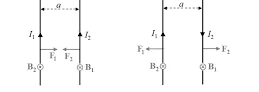#### Two thin long, parallel wires, separated by a distance d carry a current of  i  A in the same direction. They will Option 1) attract each other with a force ofOption 2) repel each other with a force ofOption 3) attract each other with a force ofOption 4) repel each other with a force ofAs we discussed in

Force between two parallel current carrying conductors -- whereinI1 and I2 current carrying two parallel wires

a-seperation between two wires

Force of attraction between wiresOption 1)

attract each other with a force ofIncorrect option

Option 2)

repel each other with a force ofIncorrect option

Option 3)

attract each other with a force ofCorrect option

Option 4)

repel each other with a force ofIncorrect option

#### Vakul